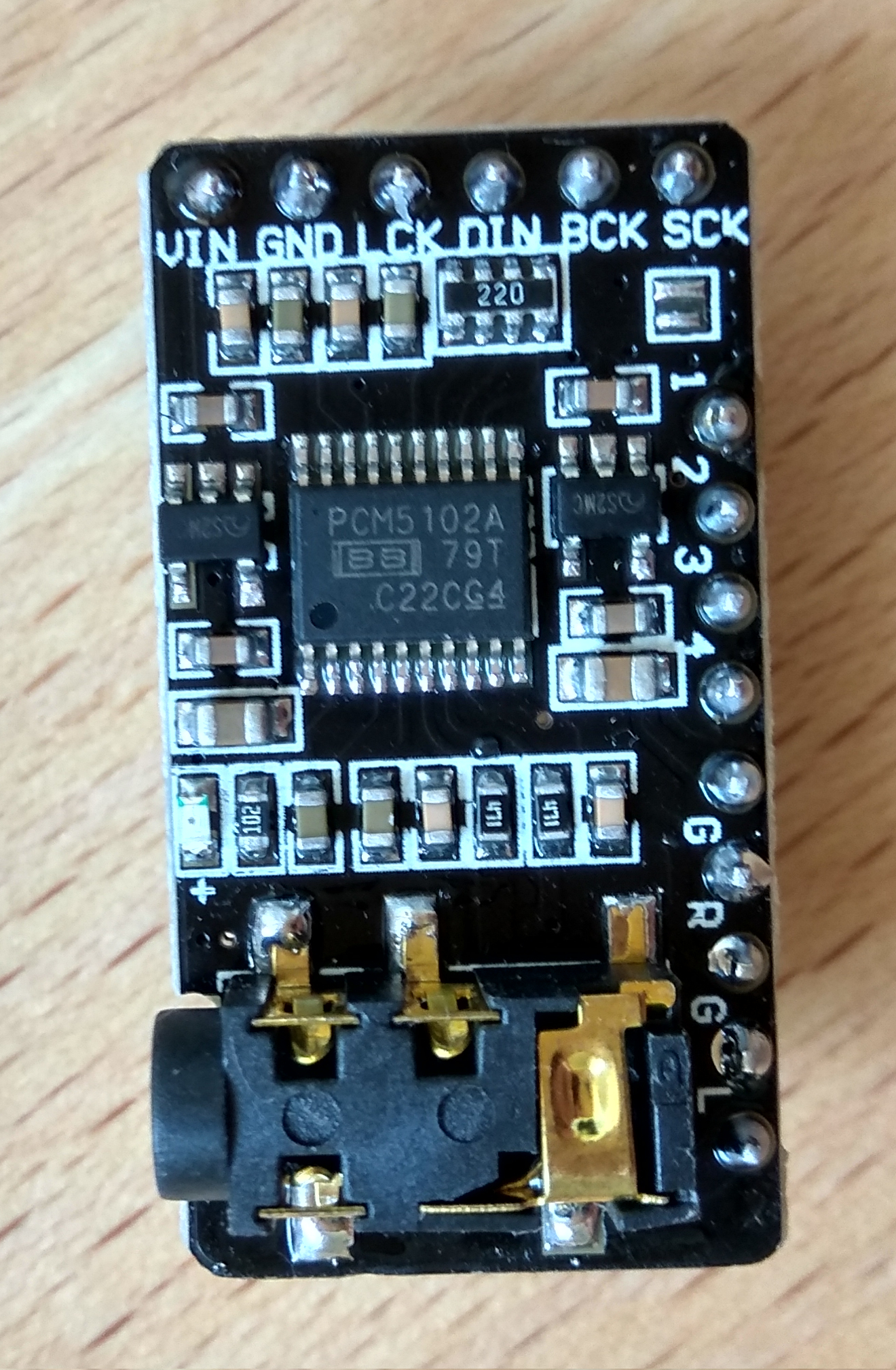# PCM Audio on ESP8266 using the PCM5102 Chip

Posted on Thu 22 August 2019 in ESP8266/ESP32 View Comments

One of the projects I am currently working on is a text to speech system based on ESP8266 (or more realistically - ESP32). It will utilize the CMU Flite speech synthesis library. But a way to play back the synthesized waveform is also required.

For this propose, I will use a PCM5102 chip that will convert digital data sent from the ESP8266 over I2S to analog signal that drive the connected speaker.ESP2866 has a hardware implemented I2S, so specific pins are required. Here is the table showing the connections I have made.

ESP8266 Name ESP8266 IO PCM5102 Pin
MTDO IO 15 BC(L)K
U0RXD IO 3 DIN
GPIO2 IO 2 L(R)CK
Connect to 3.3V VIN
Connect to GND GND

If you want to use the other pins which control certain aspects of the chips behavior, you can refer to the data-sheet. I did not connect any other pin on the breakout board of the PCM5102.

The full I2S protocol is explained on wikipedia better than anything I could write. Here are few bullets to help you understand the code which appear later:

• Data words are 16 bits two's complement.
• Each data word represents the magnitude of the wave front at specific point in time (this is called PCM)
• There are two data words for each sample representing left and right channels.
• The sample rate is, as the name suggests, number of samples (and not words) per second.
• Here, I will not utilise stereo, so each sample's left and right channels will be identical.

Ok, so first let's create 512 samples of a sine array using a python script:

```import matplotlib.pylab as plt
import numpy as np

def chunks(l, n):
"""Yield successive n-sized chunks from l."""
for i in range(0, len(l), n):
yield l[i:i + n]

SAMPLES = 512

x = np.linspace( 0, 2.0*np.pi, SAMPLES)
y = list( (int(a) if a >= 0 else int(2**16 + a) for a in  (np.sin(x)) * 2**15 ) )

for row in chunks(y, 8):
print ("".join(("{0:#0{1}x}, ".format(word,6) for word in row)))

plt.plot(x, y)
plt.show()
```

The above script prints the samples in a format suitable for embedding into a C source file.Few things to notice:

• The graph does not look like a sinus. This is because we are looking at unsigned values which represent signed data. So for example 0x8000 is actually -32768.
• The start of the wave is at the value of 0, which represents the diaphragm of the speaker at resting position. Other values would mean a sudden jump to the requested position, which will add a little bit of noise.
• There is no no intention to use stereo. The same values will be sent to both channels. Thus the "samples" are single data words and not pairs.

Using this data it will be possible to generate sine waves of various frequencies (also known as pitches).

The ESP8266 implementation of Arduino environment has a built in library for sending I2S data. All the function signatures can be found in i2s.h.

In the setup function, I set up the I2S to use 44100 Hz sample rate and the CPU to run at 160 MHz:

```#include "ESP8266WiFi.h"
#include "i2s.h"
#include "i2s_reg.h"

#define RATE 44100

void setup() {
system_update_cpu_freq(160);

i2s_begin();
i2s_set_rate(RATE);
}
```

On the ESP8266, I created a function that is similar to Arduino's tone function. But this one is not a puny, square wave, piezzo buzzer driver:

```#define SINE_SAMPLES 512

int16_t sine[SINE_SAMPLES] = { ... } // Paste here the generated samples from the python script

/**
* Outputs a sine waveover I2S of the requested frequency for the
* requested duration of milliseconds.
*/
void writeNote(uint32 freq, uint32 duration)
{
if (freq == 0 || duration == 0) {
return;
}
// Number of samples required for the requested duration
uint32 totalSamples = (RATE * duration) / 1000;

// Number of samples in full period of a sine wave for that particular frequency
uint32 samplesPerPeriod = RATE / freq;

// Round down total samples to an integer number of sine periods
totalSamples = (totalSamples / samplesPerPeriod) * samplesPerPeriod;

for (uint32 i = 0; i < totalSamples; i++) {
uint32 sampleIdx = map(i % samplesPerPeriod, 0, samplesPerPeriod, 0, SINE_SAMPLES);
i2s_write_lr( sine[sampleIdx],  sine[sampleIdx]);
}

delay(50);
}
```

You can hear in the following video, the tune of star wars played using `writeNote` function. The full source of the Arduino sketch can be found here.

Next thing I will do is playing back WAV files. Stay tuned ;)